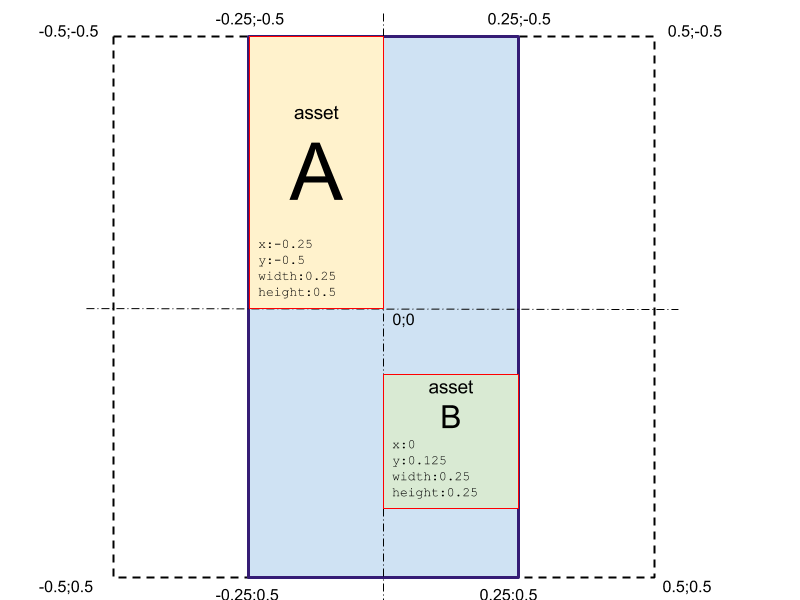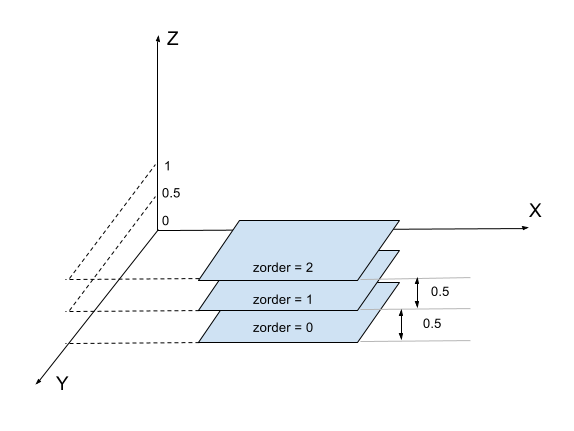# Assets positioning

For asset we have following attributes:

attribute description range
x left position of the marker’s top-left corner [-0.5:0.5]
y top position of the marker’s top-left corner [-0.5:0.5]
width asset’s width [0:1]
height asset’s height [0:1]

Assets size and position values are relative to marker’s longest size.

That’s if we have a marker `width=200px` and `height=400px` all the assets’ attributes will be relative to marker’s height (`400px` in our case).Here are some helpful formulas (W-markers width, H-marker’s height, w-asset’s width, h-asset’s height):

Position x y
top left corner of the marker x = -0.5 * min(W/H, 1) y = -0.5 * min(H/W, 1)
top right corner of the marker x = 0.5 * min(W/H, 1) y = 0.5 * min(H/W, 1)
bottom left corner of the marker x = -0.5 * min(W/H, 1) y = -0.5 * min(H/W, 1)
bottom right corner of the marker x = 0.5 * min(W/H, 1) y = 0.5 * min(H/W, 1)

### Mobile side positioning¶

#### Automatic z coordinate calculation¶

We have a problem for 2D assets with rendering assets with the same z coordinate (assets blinking). To avoid this we’ve decided to calculate z coordinate automatically by adding 0.5 to next asset in a set (ordered by zorder).

Note: This works for all 2D assets without is_3d_context and is_vertical flags.Vertical 2D assets (any NON 3D asset with is_vertical==1) For those assets z coordinate calculated front end side using following formula:

``````z = 320 * (absolute(sinus(alpha)) * [asset height in px]/2 + [altitude in px]) / [marker width in px]
``````
where altitude is a distance (in pixels) between marker and bottom of the asset

Back altitude calculation from the z coordinate:

``````altitude = z*[marker width in px] / 320- absolute(sinus(alpha)) * [asset height in px]/2
``````

#### Mobile side rendering¶

• For 3d context asset – assets rendering by z_order (from low to high)
• For plane assets – assets rendering by z coord, from high to low
• For vertical assets – nothing changes, assets rendering by z coord, from high to low